cancel
Showing results for
Did you mean:

Fabric is Generally Available. Browse Fabric Presentations. Work towards your Fabric certification with the Cloud Skills Challenge.## Understand the Filter Context and How to Control it

Scenario:
We usually hear words like words context, filter context when creating measure. Moreover, we can often see it on the DAX Guide website. But what is that? And how can we modify it?

What is filter context?
The reason why we sometimes cannot get the ideal result when we use a measure with same formula to calculate different results is the formula will be calculated based on the current filter context. For example, I have a table with two columns, one with id and one with values, like the following.

 ID Values A 1 A 2 B 3 B 4 C 5

We use a DAX expression to figure out the result, SUM(‘Table’[Values]). We put this expression into the table visual get the following result.

 ID Values A 3 B 7 C 5 Total 15

So let's see how it works during this process.
For A row, it's probable to infer that it yields 3, so it must be 1+2=3. Yes, but it has more filter context than that. To be precise, the context looks like this.

 ID Values A 1 A 2

So is B row, its context is:

 ID Values B 3 B 4

For C row, so think about it, how is Total row is calculated? 3+7+5 or 1+2+3+4+5? According to the above procedure, it should be 1+2+3+4+5. So its context should be the whole table.

 ID Values A 1 A 2 B 3 B 4 C 5

Remember that it is the table that contains all columns, including other tables with which this table is related, (but the cross filter direction must be from this table to that table, if not, this context will contain all table without being filtered), not just a single column [values]. With such a context, then this function tells power bi, I need to calculate [values], how to calculate, SUM. Then you get the result you want.

How to modify the filter context?
Before we talk about how to modify the context, we introduce a function that can indirectly view the context of the current line.

``````Context = CONCATENATEX('Table',[ID]&" "&[Values]," ")
``````

Note that the final join symbol is ALT+ENTER. This allows you to make line breaks and output results like a table.1. Relationship filter
Add a table named ”Dim Table” to this model without creating a relationship. Next, drag the [Group] field of the Dim Table into the table visual, and drag the measure named [Context] into it. The results of the graph can be obtained.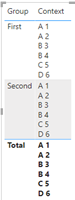Then if a one-to-many relationship is created between them based on the ID column, what will happen?

It can be seen that the corresponding grouping changes after the relationship is established. It is the related filter that occurs based on the relationship.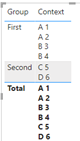2. Filter pane and slicer
We all know that measure changes dynamically when you do some filtering. So from what I just explained, you know that the measure is calculated based on the current context. So if you make some changes to the slicer, the context of each line will change, so will the measure.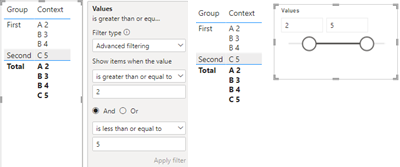From the above two pictures you can see that we set the value greater than or equal to 2 and less than or equal to 5, so the value of A 1 ,D 6 disappeared and was not shown in the [Context] measure.

3. DAX
All(), ALLSELECTED(), ALLEXPECT(), REMOVEFILTER(), these functions can remove redundant filters or keep only the filters you want. Continuing to keep the relationship between the two tables, let's compare the difference between ALL() and without ALL().

``````Context =
var _table = 'Fact Table'
return
CONCATENATEX(_table,[ID]&" "&[Values],"
")
``````
``````Context_ALL =
var _table = ALL('Fact Table')
return
CONCATENATEX(_table,[ID]&" "&[Values],"
")
``````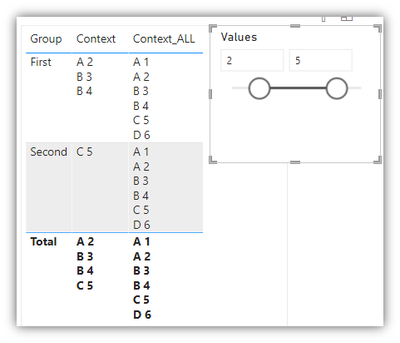Adding ALL() not only removes the filtering brought by the relationship, but also the filter brought by slicer [Values]. So, Context_ALL returns all the items in field [Values]. It seems that ALL() is telling this measure:” you need all in”. Similarly the filter brought by Slicer and Filter Pane is also rejected. ALLEXPECT(), ALLSELECTED() basically have the same effect. There are many other similar functions.

4. Custom filter context
We can customize the dynamic filtering context. Before that, let us look at a set of static ones to see how this works. A simple static table. Created via this below code:

``_table = {("test a",1),("test b",2),("test c",3)}``

And result:Then we just need to get this table dynamic next.

Let's look at the dynamic one. Now here is a question, calculate the number of ID whose [Values] is greater than the average of the group.
We first need to get all the rows belonging to the group, which we can get by using the relationship filter. So you can't use all() but you can use ALLEXPECT() to keep only the group's filter.

``var _allgrouptable = ALLEXCEPT('Dim Table','Dim Table'[Group])``

Next the average, we use the table(context) obtained by _allgrouptable to calculate the average.

``var _average = CALCULATE(AVERAGE('Fact Table'[Values]),_allgrouptable)``

Filter the table(context) obtained by relationship filter, the filter condition is [values] is greater than the above average. Calculate the number of values with COUNTROWS() and put them together.

``````Count =
var _allgrouptable = ALLEXCEPT('Dim Table','Dim Table'[Group])
var _average = CALCULATE(AVERAGE('Fact Table'[Values]),_allgrouptable)
var _context = FILTER('Fact Table',[Values]>_average)
return
COUNTROWS(_context)
``````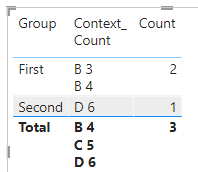Summarize:
In the end, the filter context is a table contains all the tables in your model, but not all the rows. Only those rows that are filtered by the slicer, relationship filter and DAX. So when you get a result that is not what you want, you should check if your filter context is true.

Hope this would be useful for you.

Author: Chenwu Zhu

Reviewer: Kerry Wang & Ula Huan

Top Kudoed Posts
Latest Articles